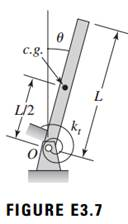### Create an Account

Home / Questions / Derive the governing equation of motion for the system shown in Figure E37 The mass moment...

# Derive the governing equation of motion for the system shown in Figure E37 The mass moment of inertia of the bar about the point O is JO and the torsion stiffness of the spring attached to the

Derive the governing equation of motion for the system shown in Figure E3.7. The mass moment of inertia of the bar about the point O is JO, and the torsion stiffness of the spring attached to the pivot point is kt. Assume that there is gravity loading.May 16 2020 View more View LessSubscribe To Get Solution